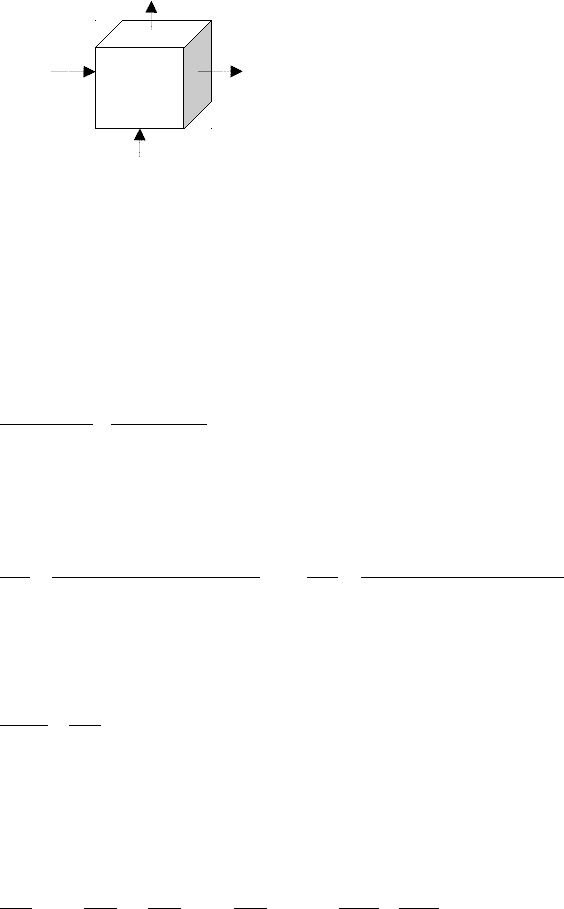# CIVL 222 Study Guide - Quiz Guide: Heat Flux, Taylor Series, Advection

90 views6 pages
School
Course
ProfessorQuiz 1 Review
Part 1: Introduction
A. Numerical Methods
Analytical Methods
Can be used to solve many algebraic expressions as well as some ODEs and PDEs
Advantages: Fast (computer time), exact; no error introduced by the solution method
Disadvantages: limited to certain physical conditions eg: homogeneous material, simple geometry
Numerical Methods
Can be used to solve a greater variety of problems
Advantages: not limited by physical conditions eg: complex geometry, heterogeneous condition
Disadvantages: approximate solution, CPU time can be large
B. Partial Dierential Equations
Order: the order of the highest order partial derivative in the equation
Linearity: is linear if the coefficients depend on the independent variable (x,y) and not the dependent
variable u
2u
∂ x2+2xy 2u
∂ y2+u=1linear 2u
∂ x2+xu ∂ u
y =x → nonlinear
Classication – 2nd Order
The general form of a second order PDE is:
A2u
∂ x2+B2u
x ∂ y +C2u
∂ y2+D∂ u
∂ x +A∂ u
∂ y +Fu+G=0
The values of A, B, C can be used to further classify this PDE based on the sign of the solution to the
equation B2 – 4AC
Elliptic if < 0, typically describe processes that are occurring at steady state (i.e. no time derivative)
Parabolic if = 0, typically describe processes where the dependent variable is changing in time with
smooth changes
Unlock document

This preview shows pages 1-2 of the document.
Unlock all 6 pages and 3 million more documents.

Already have an account? Log inqx
qy
qx+x
xz
y
Hyperbolic if > 0, also describe changes in time, but the disturbance propagates with finite speed
where sharp fronts are maintained (i.e. waves do not loose height)
Part 2: Derivation of PDEs
A. Elliptic Equations
Steady state (not changing in time) temperature distribution in 2D, ex. flow of heat through an element:
The steady state energy balance equation would be (with qx flowing through yz, qy through xz):
qx y ∆ z+qy x ∆ z=qx+∆ x y ∆ z +qy+∆ y ∆ x z
Can divide through by volume xyz and group the terms:
qxqx+∆ x
∆ x +qyqy+∆ y
∆ y =0
Recall that the definition of a derivative is
∂ u
∂ x =u
(
x+∆ x , y
)
u(x , y)
∆ x u
∂ x =u
(
x , y
)
u
(
x+∆ x, y
)
∆ x
Therefore the new expression for the energy balance equation is:
∂ q
∂ x ∂ q
y =0
We now have an equation for heat flux q, we can proceed further by replacing q with the equation for heat
flux to receive the true equation:
∂ x
(
K∂ T
∂ x
)
∂ y
(
K∂ T
∂ y
)
=02T
∂ x2+2T
∂ y2=0
qy+yBased on the flow in the x and y direction, we can write:
Accumulation = In – Out +/- Source Sink
Since steady state Accumulation = 0
Assume no source sink, therefore In = Out
Unlock document

This preview shows pages 1-2 of the document.
Unlock all 6 pages and 3 million more documents.

Already have an account? Log in

# Get access

Grade+
\$10 USD/m
Billed \$120 USD annually
Homework Help
Class Notes
Textbook Notes
40 Verified Answers
Study Guides
1 Booster Class